Select Page

# Introduction to Financial Statements

In this video series the financial statements are introduced in the context of building a financial model. The objective is not simply to explain what they are, but to demonstrate how they link to each other. These links are critical to the mechanical process of building a three statement model, and for this reason I would encourage you to review this series even if you have had previous exposure to the three primary financial statements. This series is broken down into the chapters that follow:

The Accounting Equation (LINK) (PDF NOTES)
The accounting equation is the single most important concept in accounting, and it is instrumental in developing a more thorough understanding of financial statements.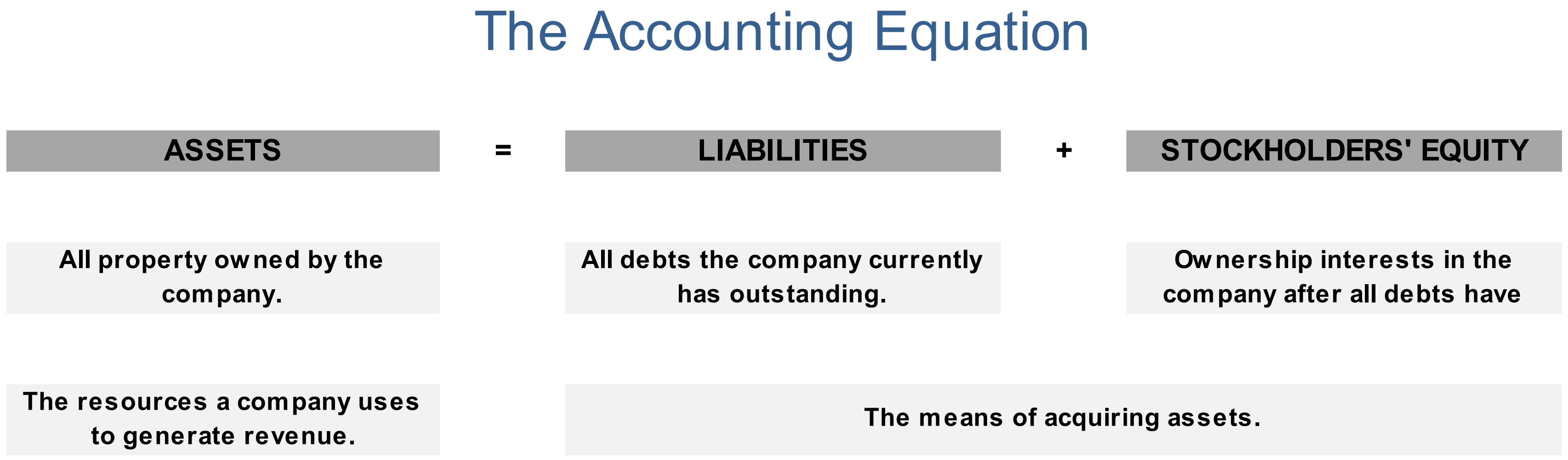This video explains how the accounting equation is relevant in building financial models. It also provides a cursory overview of double-entry bookkeeping, which is the system most commonly employed by businesses to record financial information. The video concludes by pointing out that the balance sheet is simply a more formal presentation of the accounting equation.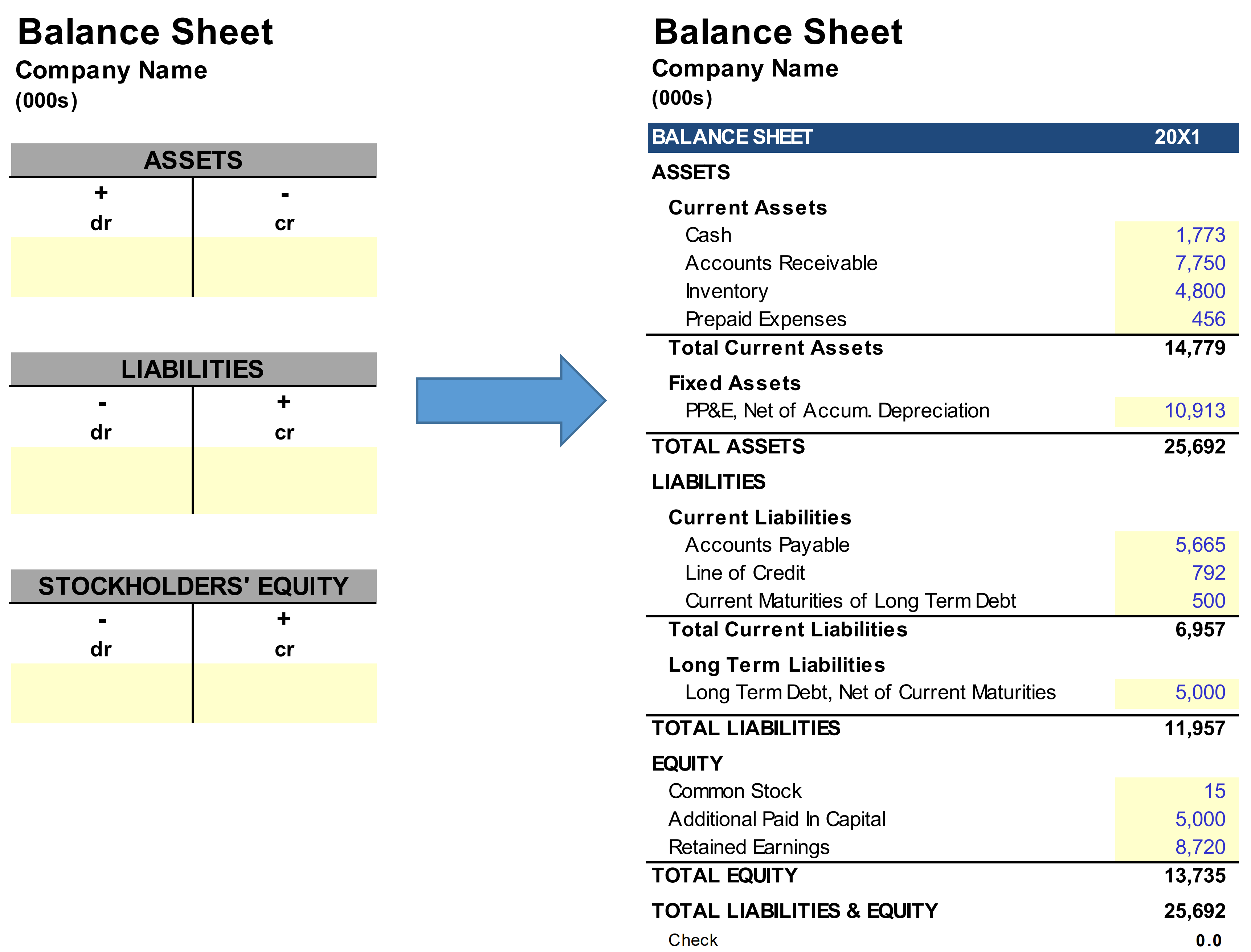The Balance Sheet (LINK(PDF NOTES)
This video introduces the balance sheet by asking you to imagine the resources you would require to operate a business, and then to visualize the means of securing these resources. By reorganizing these variables you can see how all of them fall into one of three categories: Assets, Liabilities and Stockholders’ Equity. The video then emphasizes that the balance sheet details a company’s financial position at any given time, whereas the income statement and cash flow statement detail the economic activity that has elapsed between periods.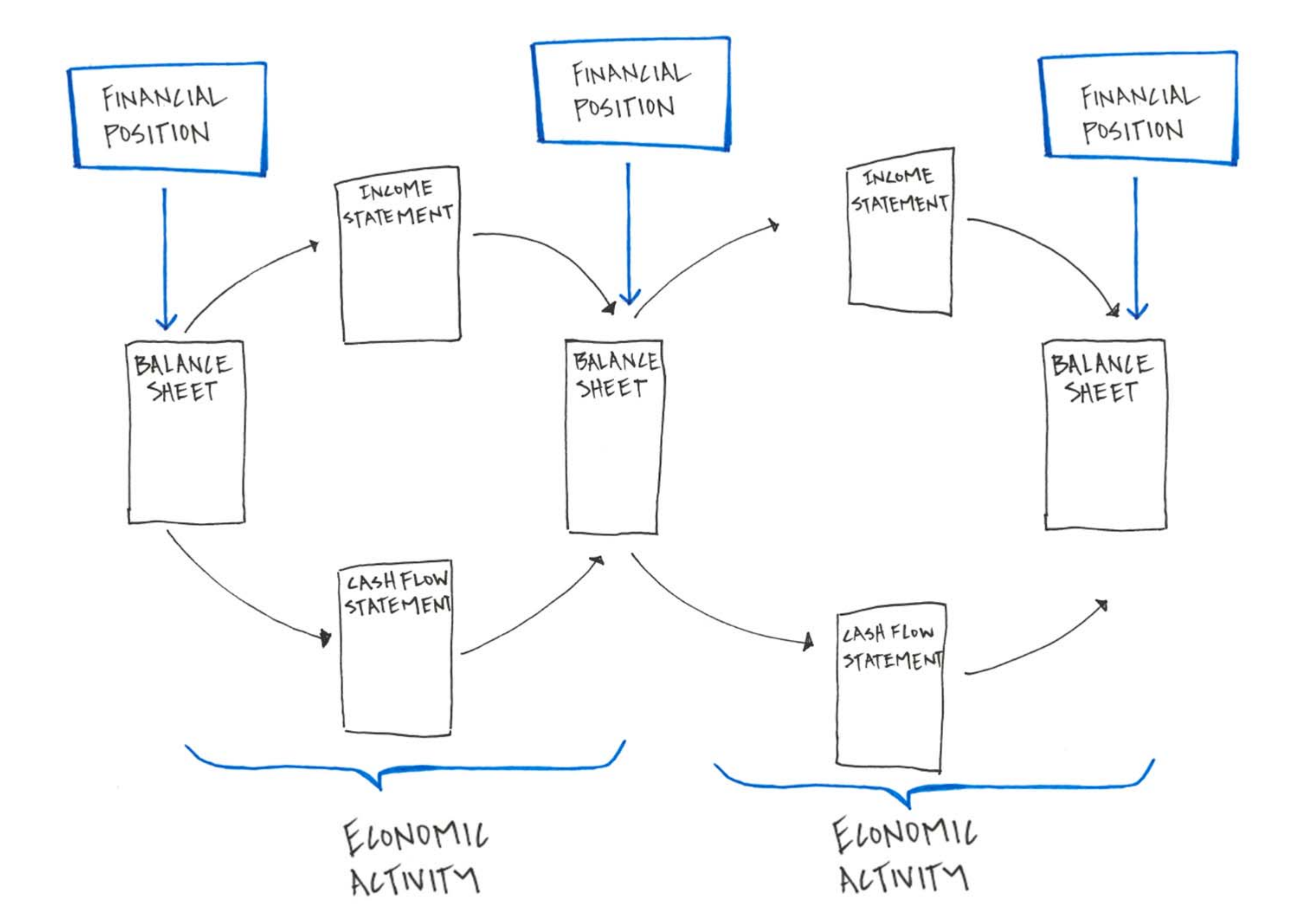The instruction concludes by highlighting relationships between the balance sheet and other financial statements that are important to keep in mind as you build financial models.

The Income Statement (LINK) (PDF NOTES)
This video introduces the income statement by starting with the most concise presentation and expanding on the number of line items. After this introduction the accounting equation is revisited to help illustrate how the balance sheet and income statement relate to one another (green triangle in the image that follows – the most significant relationship highlighted is that stockholders’ equity grows with net income).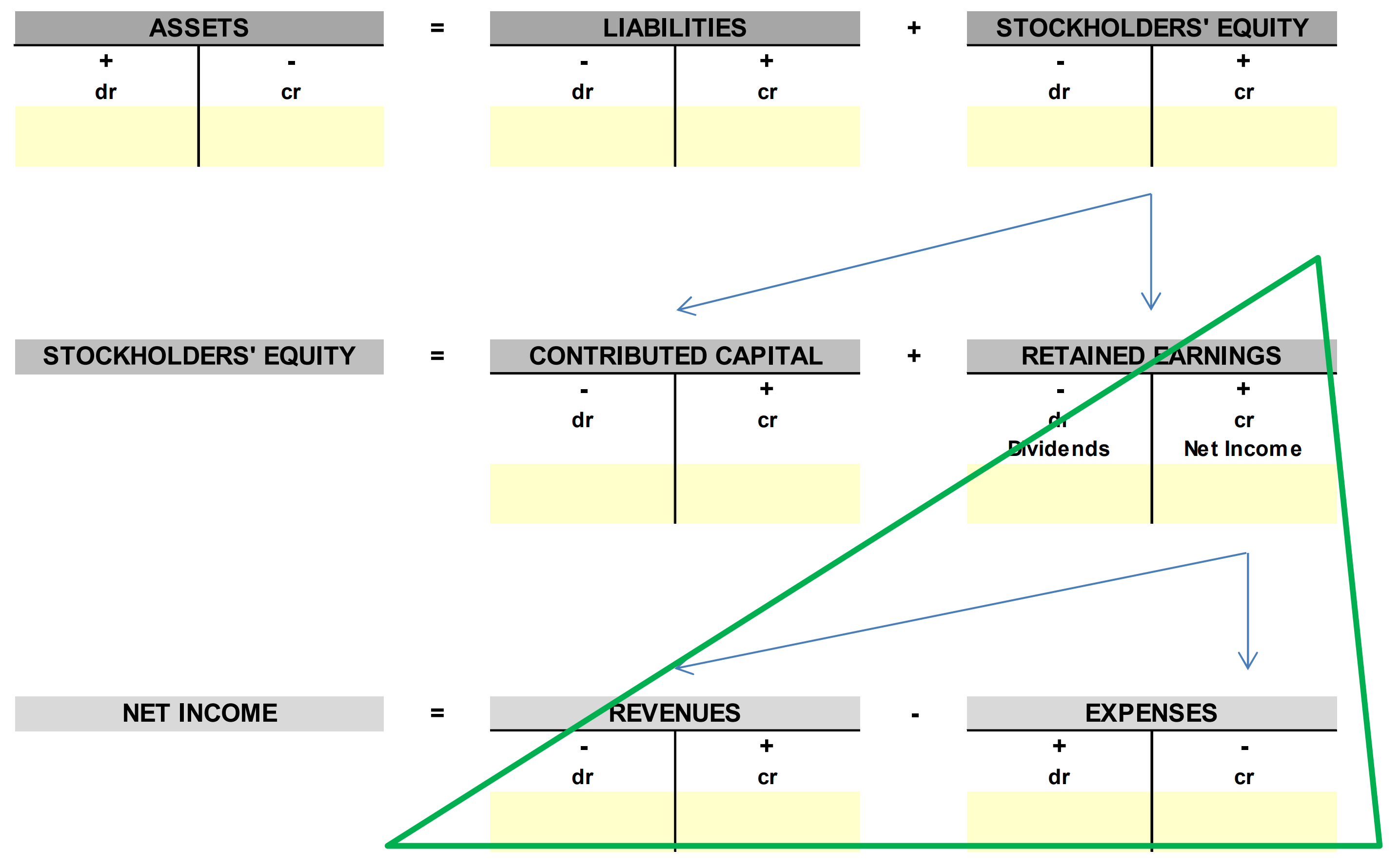Next the purpose of the income statement is defined and two important accounting concepts are introduced: the Matching Principle and Depreciation. The video concludes by highlighting the relationship between the income statement and the cash flow statement, and highlighting the difference between an accrual basis of accounting and a cash basis of accounting.

Cash Flow Statement (LINK) (PDF NOTES)
This video introduces the cash flow statement, which is possibly the most straightforward of the three primary financial statements. Whereas both the income statement and balance sheet reflect an accrual basis of accounting, the cash flow statement starts with net income and translates the economic activity of the firm from an accrual basis to a cash basis. To demonstrate the difference, the video walks through the purchase of a large piece of equipment on both an accrual and cash basis providing visuals to help cement these concepts. The video also goes into detail on the three different types of cash inflows and outflows: Cash Flow from Operating Activities, Cash Flow from Investing Activities and Cash Flow from Financing Activities. The video concludes by explaining the significance of measuring cash and why GAAP measures of profitability in isolation are not sufficient to determine a business’s financial condition.

With the introduction of all three financial statements complete, the video then explains how the three financial statements work together to maintain the accounting equation. The first relationship highlighted is that the cash balance calculated on the cash flow statement links to cash on the balance sheet (see arrow on left-hand side of the image below). In this way the cash flow statement adjusts the asset side of your balance sheet in each consecutive accounting period. As a reminder, the video then shows that net income adjusts the equity account (retained earnings) in each accounting period (see arrow on right-hand side of the image below).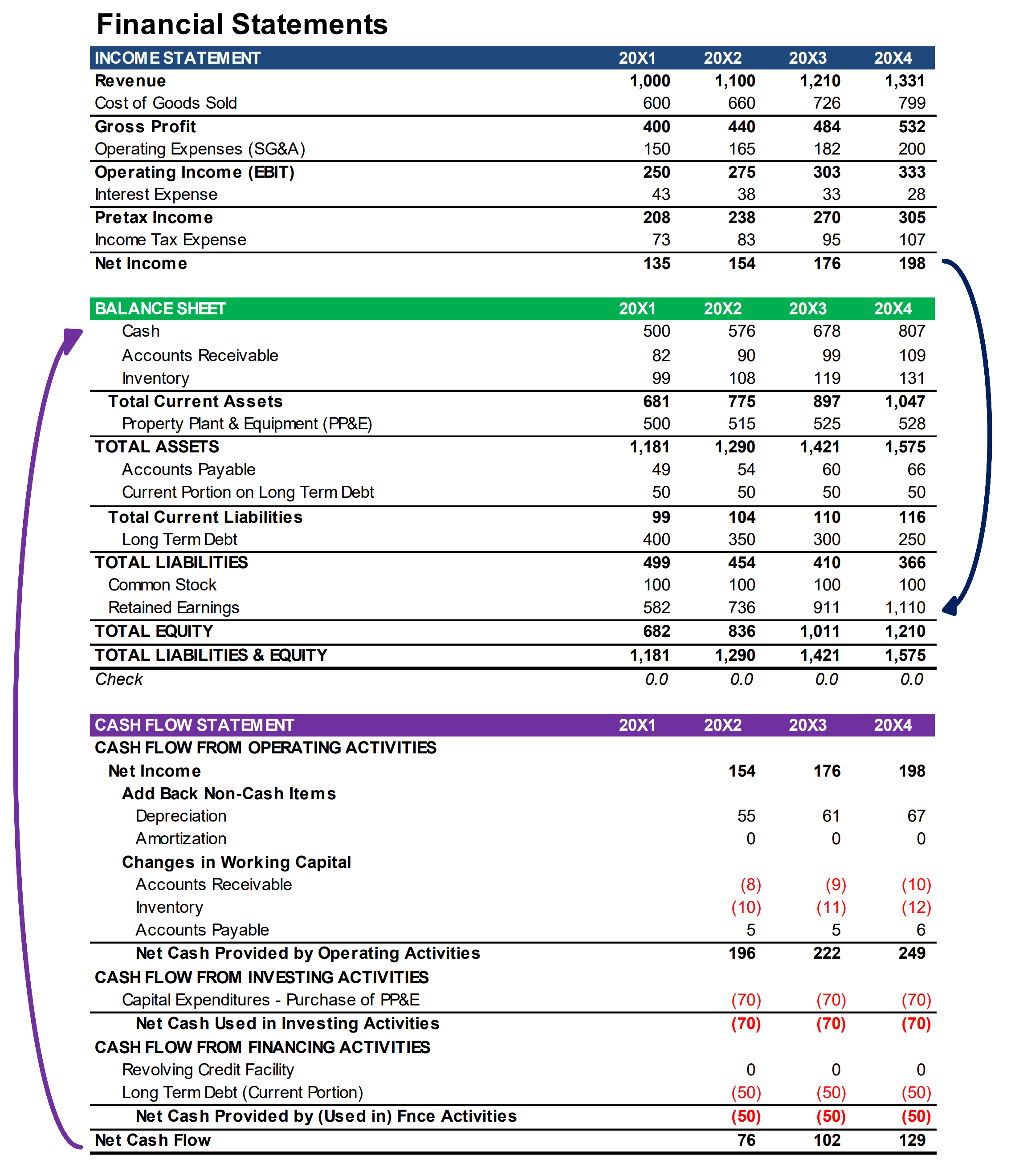With that in mind, recall that the balance sheet is just a formal presentation of the accounting equation. If the cash flow statement adjusts the left-hand side of the equation, or assets, by the companies cash flow in that period, and the income statement adjusts the right-hand side of the equation, or stockholders’ equity, by net income, then the cash flow statement, which starts with net income, is making adjustments so that the accounting equation holds true. And that is how the accounting equation is balanced in financial models, and therefore how the balance sheet is balanced in financial models.

Handwritten Quiz
The video series concludes with a handwritten quiz. There is only so much that can be accomplished with multiple choice and fill-in-the-blank quizzes. These formats are helpful because they provide the ability to immediately show results, which is critical to the learning process. But between the two, research shows that fill-in-the-blank is more effective because it requires retrieving the answer from memory without the ease of selecting from a list of options. It follows, that writing answers in longhand takes this benefit a step further.

Once you have completed this video series, ASM suggests advancing to the Integrating Financial Statements video series.